# When Does 1756 Look a Little Like π?

### Today’s Puzzle:

Here are 1756 tiny squares. Solve for n and you will know which centered pentagonal number 1756 is. You could also count the pentagons from the center outward.What triangular number multiplied by 5 is one less than 1756?

How do you pronounce pentagonal? Here’s a quick video on its correct pronunciation:

Confession: I had been mispronouncing all of those terms, but not anymore!

### Factors of 1756:

• 1756 is a composite number.
• Prime factorization: 1756 = 2 × 2 × 439, which can be written 1756 = 2² × 439.
• 1756 has at least one exponent greater than 1 in its prime factorization so √1756 can be simplified. Taking the factor pair from the factor pair table below with the largest square number factor, we get √1756 = (√4)(√439) = 2√439.
• The exponents in the prime factorization are 2 and 1. Adding one to each exponent and multiplying we get (2 + 1)(1 + 1) = 3 × 2 = 6. Therefore 1756 has exactly 6 factors.
• The factors of 1756 are outlined with their factor pair partners in the graphic below.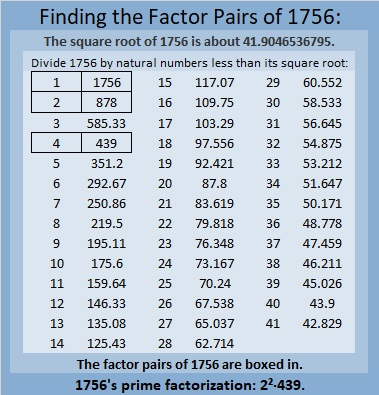### More About the Number 1756:

The centered pentagon above doesn’t really look like π, so when does 1756 look a little bit like π? Pi day in America is next week, so this is a time-sensitive question.

Actually,1756 looks a little like 10π in base 24. You see 1756₁₀ is the same as 314₂₄.
Why? Because 3(24²) + 1(24) + 4(1) = 1756.

1756₁₀ also is the same as 1024₁₂.
Why? Because 1(12³) + 0(12²) + 2(12) + 4(1) = 1756.
I think that’s cool because 2¹º = 1024.

1756 is the difference of two squares:
440² – 438² = 1456.

# 1755 Searching for Prime Decades

### Today’s Puzzle:

Charles Sanders got me thinking about prime decades and how to find them. What a great puzzle for me to work on!

A prime decade is a set of four prime numbers that look the same except for their last digit.

The first decade has 4 prime numbers, 2, 3, 5, 7, but it is NOT a prime decade because 1 and 9 are not prime numbers. 5, 7, 11, 13 is the only prime quadruplet that is not also a prime decade. The first prime decade is 11, 13, 17, 19.

Could 1751, 1753, 1757, and 1759 form a prime decade? Since 1755 is divisible by 15, none of those other four odd numbers can be divisible by 3 or 5, so it is worth looking into if they are all prime numbers. We don’t have to test all four numbers, however, we only need to test 1759 using modular arithmetic. If we note the remainder when we divide 1759 by each prime number from 7 to 41, we will know. (We only have to go to 41 because √1759 < 43, so we don’t need any prime number divisor greater than or equal to 43.)

Will any remainder be a red flag? Yes, if any remainder is 0, 2, 6, or 8, then one of those four odd numbers won’t be prime. Also, if we divide 1759 by 7 and get a remainder of 1, then 1758 and 1751 will both be divisible by 7, and we won’t have a prime decade.

I did the divisions and everything looked great except
1759 ÷ 7 has a remainder of 2, so 1759 – 2 or 1757 is divisible by 7, and
1759 ÷ 17 has a remainder of 8, so 1759 – 8 or 1751 is divisible by 17.

In general, to find prime decades we only need to test the last number of the decades and we only need to test decades if the last number minus 4 is divisible by 15. I made a chart showing only those last numbers, and they appear in the first column. The top two rows are the prime number divisors and their squares. We will divide the last number in the decades by the prime numbers whose square is less than that last number. The remainders in pink are red flags. That would be a 1 in the 7’s column, and 0, 2, 6, or 8 in any column. Any of those would disqualify a decade from being a prime decade. 🙁

The green last numbers (in the first column) show the last number of actual prime decades. 🙂
The yellow last numbers have only ONE divisor that disqualifies the decade. 😐

What are the prime decades in this first millennia?As you can see, decades ending in 19, 109, 199, and 829 are all prime decades. This chart can also be useful in finding prime triplets because at least two of the prime numbers in a prime triplet whose first prime is greater than 10 must come from a decade whose middle number is divisible by 15. If a decade has two prime triplets in it that meet that qualification, it is also a prime decade.

In the next graphic, we see that the decades ending in 1489 and 1879 are prime decades. Here we also test the decade in which our post number, 1755, appears. We see 1759 has remainders of 2 and 8, confirming that 1757 and 1751 are not prime numbers.There is only one prime decade, 2081, 2083, 2087, 2089, in the next set of decades, and only one other decade that even came close to being a second one.There are two prime decades in the next group and several close calls:None in the next group, but there are several decades that ALMOST qualified.There’s a prime decade is in this group as well as a handful of decades that almost qualified.In this next group, there are only two decades that even came close to qualifying.The next two millennia have no prime decades but they do have several decades that came close to qualifying.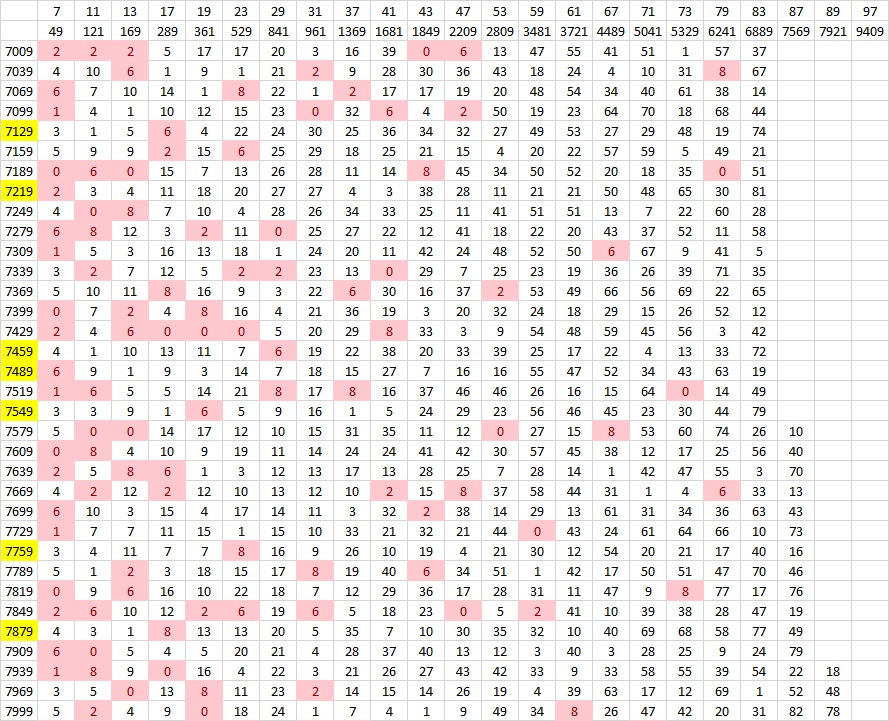Finally, we get another prime decade in this next group! (We would have had one right away if only 9047 wasn’t divisible by 83.)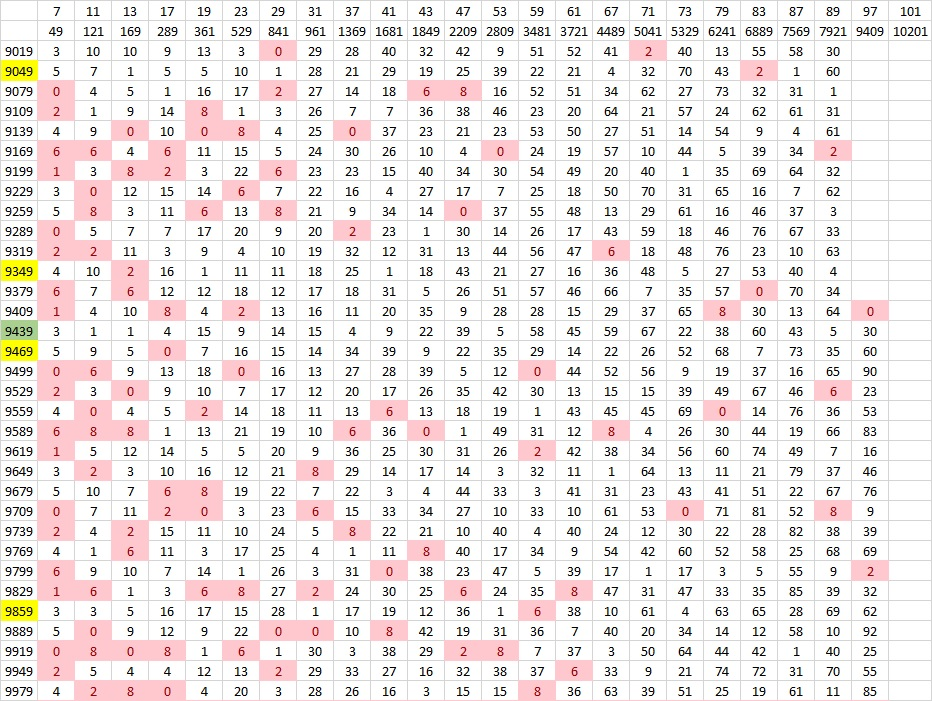I could go on literally forever, but I’ll stop now. Instead, I’ll write a little more about the post number, 1755.

### Factors of 1755:

• 1755 is a composite number.
• Prime factorization: 1755 = 3 × 3 × 3 × 5 × 13, which can be written 1755 = 3³ × 5 × 13.
• 1755 has at least one exponent greater than 1 in its prime factorization so √1755 can be simplified. Taking the factor pair from the factor pair table below with the largest square number factor, we get √1755 = (√9)(√195) = 3√195.
• The exponents in the prime factorization are 3,1 and 1. Adding one to each exponent and multiplying we get (3 + 1)(1 + 1) (1 + 1) = 4 × 2 × 2 = 16. Therefore 1755 has exactly 16 factors.
• The factors of 1755 are outlined with their factor pair partners in the graphic below.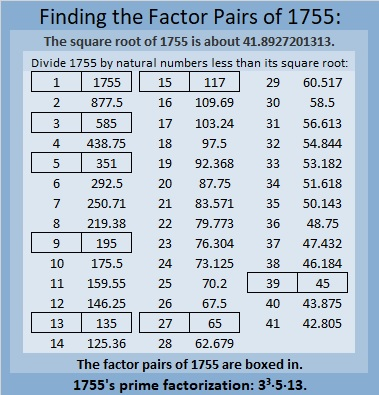### More About the Number 1755:

1755 is the hypotenuse of FOUR Pythagorean triples:
432-1701-1755, which is 27 times (16-63-65)
675-1620-1755, which is (5-12-13) times 135,
891-1512-1755, which is 27 times (33-56-65), and
1053-1404-1755, which is (3-4-5) times 351.

# 1754 and a Level 2 Puzzle

### Today’s Puzzle:

Can you write each number from 1 to 12 in the first column and again in the top row so that the products of the numbers you write are the given clues?### Factors of 1754:

• 1754 is a composite number.
• Prime factorization: 1754 = 2 × 877.
• 1754 has no exponents greater than 1 in its prime factorization, so √1754 cannot be simplified.
• The exponents in the prime factorization are 1 and 1. Adding one to each exponent and multiplying we get (1 + 1)(1 + 1) = 2 × 2 = 4. Therefore 1754 has exactly 4 factors.
• The factors of 1754 are outlined with their factor pair partners in the graphic below.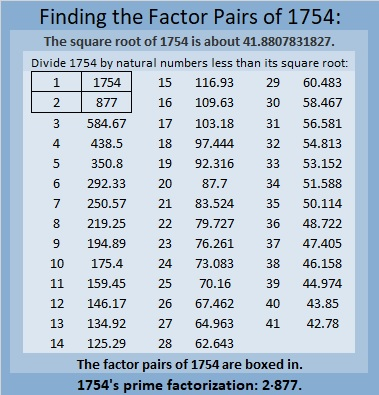### More About the Number 1754:

1754 is the sum of two squares:
35² + 23² = 1754.

1754 is the hypotenuse of a Pythagorean triple:
696-1610-1754, calculated from 35² – 23², 2(35)(23), 35² + 23².
It is also 2 times (348-805-877).

# 1753 What Is the Slope of the Driveway?

### Today’s Puzzle:

With so much snow all around it is easy to think about summer. Last summer my family and I stayed at a magnificent Airbnb. There was more than enough room for my husband and me, our children and their spouses, and our grandchildren (32 people in total).

The owners of the Airbnb wrote that the best views come from homes with steep driveways. I will share some of those views later in the post. But just how steep was the driveway? I took a picture and added a grid so you can calculate the slope. Notice that the driveway zigzags. You can estimate where the front tire of the van parked at the top of the driveway hits the pavement to calculate the slope of the top part of the driveway.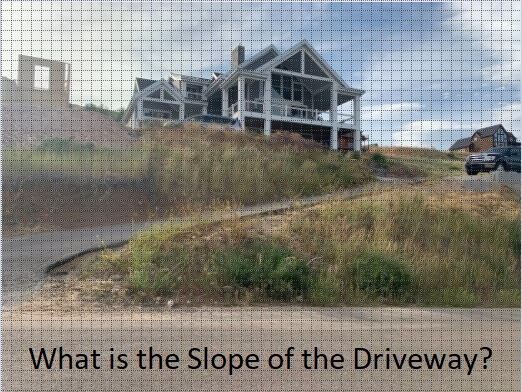Here’s the top half of the driveway:

###The beautiful view from the top of the driveway:

###The next three pictures are gorgeous views from inside the house or from a balcony:

###The views definitely made the steep driveway worth it!

Since this is my 1753rd post, I’ll write a little about that number.

### Factors of 1753:

• 1753 is a prime number.
• Prime factorization: 1753 is prime.
• 1753 has no exponents greater than 1 in its prime factorization, so √1753 cannot be simplified.
• The exponent in the prime factorization is 1. Adding one to that exponent we get (1 + 1) = 2. Therefore 1753 has exactly 2 factors.
• The factors of 1753 are outlined with their factor pair partners in the graphic below.How do we know that 1753 is a prime number? If 1753 were not a prime number, then it would be divisible by at least one prime number less than or equal to √1753. Since 1753 cannot be divided evenly by 2, 3, 5, 7, 11, 13, 17, 19, 23, 29, 31, 37, or 41, we know that 1753 is a prime number.

### More About the Number 1753:

1753 is a powerful sum:
2¹º + 3⁶ = 1753.

Since both of those exponents are even, 1753 is also the sum of two squares:
32² + 27² = 1753.

1753 is the hypotenuse of a Pythagorean triple:
295-1728-1753, calculated from 32² – 27², 2(32)(27), 32² + 27².

Here’s another way we know that 1753 is a prime number: Since its last two digits divided by 4 leave a remainder of 1, and 32² + 27² = 1753 with 32 and 27 having no common prime factors, 1753 will be prime unless it is divisible by a prime number Pythagorean triple hypotenuse less than or equal to √1753. Since 1753 is not divisible by 5, 13, 17, 29, 37, or 41, we know that 1753 is a prime number.

# 1752 So Many Snowflakes

### Today’s Puzzle:

A winter storm hit much of the United States today including the drought-stricken area where I live. We are so grateful for the snow, but also grateful we could stay home all day. Driving in this much snow is not much fun.

This snowflake mystery-level puzzle might have a couple of tricky clues, but I’m confident that you’ll still find it easier than clearing a couple of feet of snow off a driveway! Just write the numbers 1 to 12 in both the first column and the top row so that those numbers and the given clues make a multiplication table.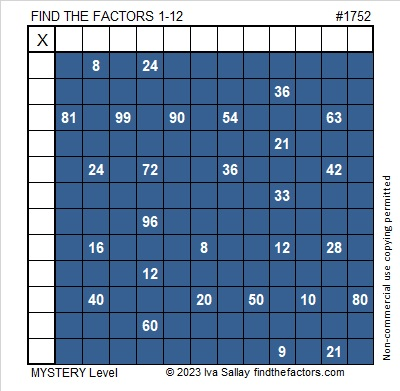Here’s the same puzzle without any added color: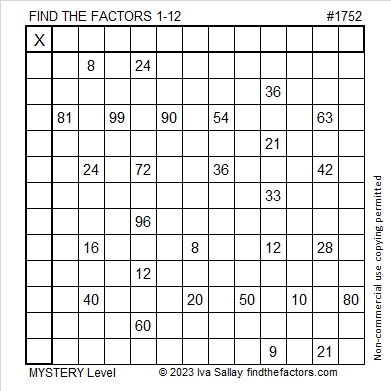### Factors of 1752:

• 1752 is a composite number.
• Prime factorization: 1752 = 2 × 2 × 2 × 3 × 73, which can be written 1752 = 2³ × 3 × 73.
• 1752 has at least one exponent greater than 1 in its prime factorization so √1752 can be simplified. Taking the factor pair from the factor pair table below with the largest square number factor, we get √1752 = (√4)(√438) = 2√438.
• The exponents in the prime factorization are 3,1 and 1. Adding one to each exponent and multiplying we get (3 + 1)(1 + 1) (1 + 1) = 4 × 2 × 2 = 16. Therefore 1752 has exactly 16 factors.
• The factors of 1752 are outlined with their factor pair partners in the graphic below.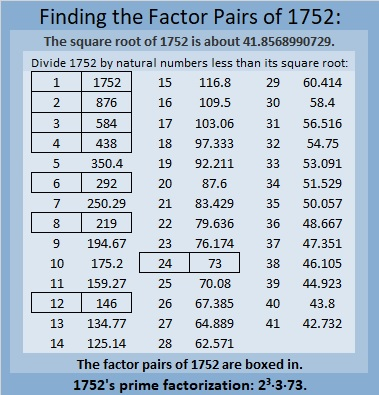### More About the Number 1752:

1752 is the hypotenuse of a Pythagorean triple:
1152 1320 1752, which is 24 times (48-55-73).

1752 is the difference of two squares in FOUR different ways:
439² – 437² = 1752,
221² – 217² = 1752,
149² -143² = 1752, and
79² – 67² = 1752.

1752 is a palindrome in some other bases:
It’s A4A in base13 because 10(13²) + 4(13) + 10(1) = 1752,
it’s 4G4 in base19 because 4(19²) + 16(19) + 4(1) = 1752, and
it’s 2K2 in base 25 because 2(25²) + 20(25) + 2(1) = 1752.

# 1751 A Pythagorean Triple Puzzle

### Today’s Puzzle:

Even though they don’t look like it, the fourteen triangles in today’s puzzle are all Pythagorean triple triangles that most certainly have not been drawn to scale. The marked angle is the right angle for each of triangle. The hypotenuse of each triangle is less than 100. There is a chart under the puzzle listing all possible Pythagorean triples that could be used in the puzzle. No triple will be used more than once. Use logic to figure out what the missing side lengths are. Have fun!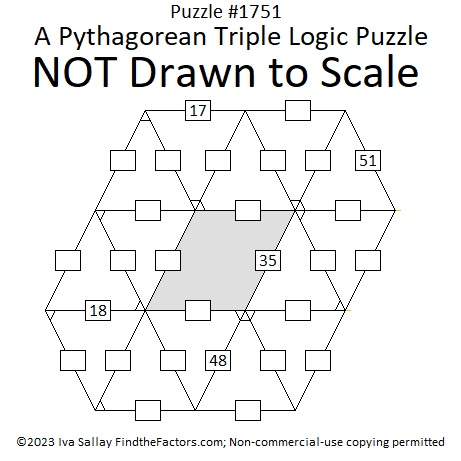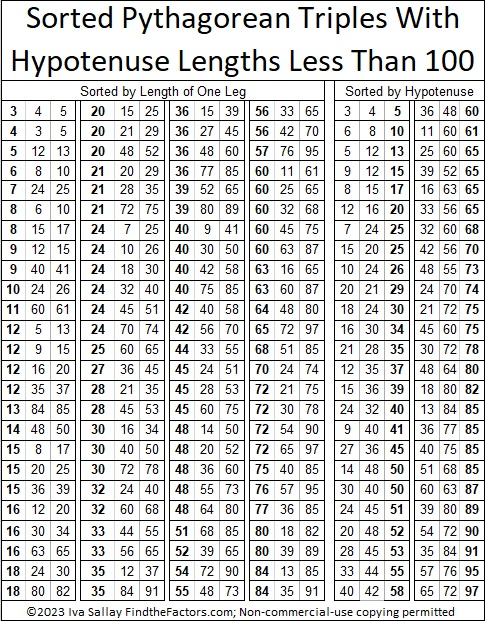### Factors of 1751:

If you remember that 51 is NOT a prime number because 3 × 17 = 51, then it isn’t difficult to recognize that 1751 also must be divisible by 17.

• 1751 is a composite number.
• Prime factorization: 1751 = 17 × 103.
• 1751 has no exponents greater than 1 in its prime factorization, so √1751 cannot be simplified.
• The exponents in the prime factorization are 1 and 1. Adding one to each exponent and multiplying we get (1 + 1)(1 + 1) = 2 × 2 = 4. Therefore 1751 has exactly 4 factors.
• The factors of 1751 are outlined with their factor pair partners in the graphic below.### More About the Number 1751:

1751 is the hypotenuse of a Pythagorean triple:
824-1545-1751 which is (8-15-17) times 103.

1751 looks interesting in some other bases:
It’s 575 in base 18 because 5(18²) + 7(18) + 5(1) = 1751,
373 base 23 because 3(23²) + 7(23) + 3(1) = 1751, and
1F1 base 35 because 1(35²) + 15(35) + 1(1) = 1751.

# 1750 and Level 1

### Today’s Puzzle:

Place the numbers 1 to 12 in the first column and also in the top row so that those numbers are the factors of the given clues.### Factors of 1750:

• 1750 is a composite number.
• Prime factorization: 1750 = 2 × 5 × 5 × 5 × 7, which can be written 1750 = 2 × 5³ × 7.
• 1750 has at least one exponent greater than 1 in its prime factorization so √1750 can be simplified. Taking the factor pair from the factor pair table below with the largest square number factor, we get √1750 = (√25)(√70) = 5√70.
• The exponents in the prime factorization are 1,3 and 1. Adding one to each exponent and multiplying we get (1 + 1)(3 + 1)(1 + 1) = 2 × 4 × 2 = 16. Therefore 1750 has exactly 16 factors.
• The factors of 1750 are outlined with their factor pair partners in the graphic below.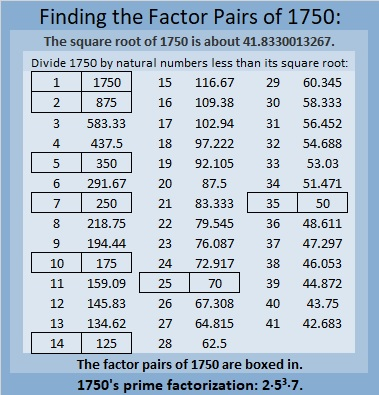### More About the Number 1750:

1750 is the hypotenuse of THREE Pythagorean triples:
490 1680 1750, which is (7-24-25) times 70,
616 1638 1750, which is 14 times (44-117-125), and
1050 1400 1750, which is (3-4-5) times 350.

1750 looks interesting in some other bases:
It’s 5050 in base 7 because 5(7³) + 5(7) = 1750, and it’s
1K1 in base 33 because 1(33²) + 20(33) + 1(1) = 1750.

# 1749 Happy Valentine’s Day!

### Today’s Puzzle:

Here’s one more Valentine’s puzzle for you to do. Enjoy!### Factors of 1749:

1749 is divisible by 3 because 7 is half of 14 so any 3-digit number made from the digits 714 in any order is divisible by 3, and of course, 9 is divisible by 3.

1749 is divisible by 11 because 1 – 7 + 4 – 9 = -11, a multiple of 11.

• 1749 is a composite number.
• Prime factorization: 1749 = 3 × 11 × 53.
• 1749 has no exponents greater than 1 in its prime factorization, so √1749 cannot be simplified.
• The exponents in the prime factorization are 1, 1, and 1. Adding one to each exponent and multiplying we get (1 + 1)(1 + 1)(1 + 1) = 2 × 2 × 2 = 8. Therefore 1749 has exactly 8 factors.
• The factors of 1749 are outlined with their factor pair partners in the graphic below.### More About the Number 1749:

1749 is the hypotenuse of a Pythagorean triple:
924-1485-1749 which is 33 times (28-45-53).

1749 is the difference of two squares in FOUR different ways:
875² – 874² = 1749,
293² – 290² = 1749,
85² – 74² = 1749, and
43² – 10² = 1749.

# 1748 A Factor Fits Valentine’s Puzzle

### Today’s Puzzle:

Can you find the one and only solution to this Factor Fits Valentine puzzle or will it give you fits?### Factors of 1748:

• 1748 is a composite number.
• Prime factorization: 1748 = 2 × 2 × 19 × 23, which can be written 1748 = 2² × 19 × 23.
• 1748 has at least one exponent greater than 1 in its prime factorization so √1748 can be simplified. Taking the factor pair from the factor pair table below with the largest square number factor, we get √1748 = (√4)(√437) = 2√437.
• The exponents in the prime factorization are 2, 1, and 1. Adding one to each exponent and multiplying we get (2 + 1)(1 + 1)(1 + 1) = 3 × 2 × 2 = 12. Therefore 1748 has exactly 12 factors.
• The factors of 1748 are outlined with their factor pair partners in the graphic below.### More About the Number 1748:

1748 is the difference of two squares in two ways:
438² – 436² = 1748, and
42² – 4² = 1748.
That means we are only 16 numbers away from the next perfect square!

# 1747 Getting a Super Bowl Ring Isn’t Easy

### Today’s Puzzle:

On Wednesday I read an article in my local paper titled From undrafted free agent to the biggest stage: Britain Covey’s ‘unique ride’ to Super Bowl LVII. It got me quite excited for today’s game. Unfortunately, between Wednesday and Sunday, Covey suffered a hamstring injury.  Getting a Super Bowl Ring certainly isn’t easy. Being able to contribute to your team’s winning the game isn’t easy either. UPDATE: Good News! He was able to make at least one play in the second quarter! Further update: Even though his team lost the game in the final minutes, this rookie played well when he was on the field. I think both teams played exceptionally well and gave us all an exciting game to watch.

Before the game, it is a mystery which team will win the game. The difficulty level of this puzzle is a mystery also.

If you look at today’s puzzle just right, I think it looks a little like a super bowl ring, but I forwarn you, it will not be easy to get this puzzle either. You will need to place the numbers from 1 to 12 both in the first column and in the top row so that the given clues are the products of the numbers you place. Logic and practice will get you there. Good Luck!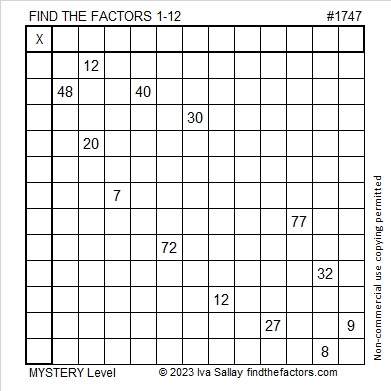You also might enjoy this next Super Bowl puzzle I saw on Twitter:

### Factors of 1747:

• 1747 is a prime number.
• Prime factorization: 1747 is prime.
• 1747 has no exponents greater than 1 in its prime factorization, so √1747 cannot be simplified.
• The exponent in the prime factorization is 1. Adding one to that exponent we get (1 + 1) = 2. Therefore 1747 has exactly 2 factors.
• The factors of 1747 are outlined with their factor pair partners in the graphic below.How do we know that 1747 is a prime number? If 1747 were not a prime number, then it would be divisible by at least one prime number less than or equal to √1747. Since 1747 cannot be divided evenly by 2, 3, 5, 7, 11, 13, 17, 19, 23, 29, 31, 37, or 41, we know that 1747 is a prime number.

### More About the Number 1747:

1747 is a palindrome in base 15.
It’s 7B7₁₅ because 7(15²) + 11(15) + 7(1) = 1747.